# 几何变换（Transforms）¶

Easygraphics（和其使用的Qt系统）支持5种基本几何变换：

• 平移（Translation）: 将图形上的各点向同一方向移动相同距离。
• 旋转（Rotation）: 图形上各点在保持相对位置固定的同时，围绕一个定点运动。
• 缩放（Scaling） : 在各轴方向上分别将图形放大(enlarge)或缩小(shrink)相应的倍数（factor）。
• 错切(Shear Mapping) : 是 一种线性映射，将形状上的所有点向一个方向推移，推移的距离与各点到与该方向平行的一条直线的距离成正比。
• 反射(Reflection): 图像通过一条直线(对称轴)变换成它的镜像。原图像上各点到对称轴的距离，和其在镜像上对应点到对称轴的距离相等。

```def draw_bus():
"""
Draw a simple bus
"""
set_color("lightgray")
rect(60, 20, 270, 150)

set_color("red")
set_fill_color("blue")

draw_circle(120, 120, 10)  # draw tyre
draw_circle(200, 120, 10)  # draw tyre
rect(80,40,250,120)

# draw window
x = 90
while x < 175 :
rect(x, 60, x+10, 70)
x += 15

# draw door
rect(220, 60, 240, 120);
line(230, 60, 230, 120);
circle(230, 90, 5);
```

```from easygraphics import *
import draw_bus

def main():
init_graph(500, 300)
draw_bus.draw_bus()
pause()
close_graph()

easy_run(main)
```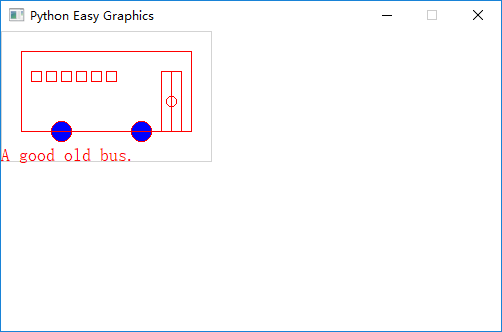## 平移（Translation）¶

```from easygraphics import *
import draw_bus

def main():
init_graph(500, 300)
translate(250, 150)
draw_bus.draw_bus()
pause()
close_graph()

easy_run(main)
```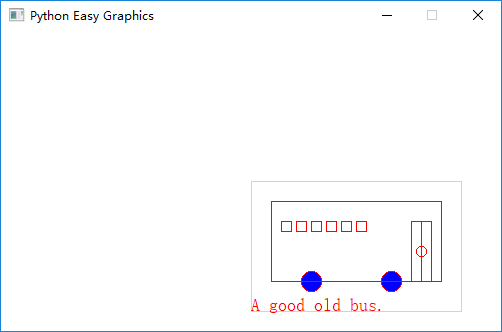## 旋转（Rotation）¶

```from easygraphics import *
import draw_bus

def main():
init_graph(500, 300)
# rotate around the (105,65)
rotate(-45, 105, 65)

draw_bus.draw_bus()
pause()
close_graph()

easy_run(main)
```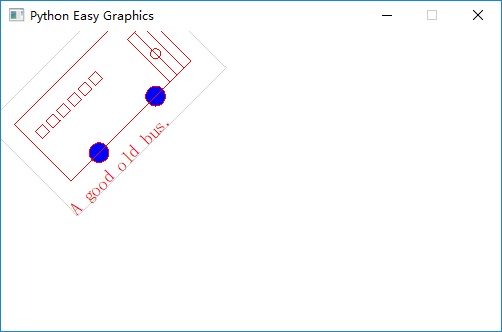## 缩放（Scaling）¶

```from easygraphics import *
import draw_bus

def main():
init_graph(500, 300)

scale(0.5, 2)
draw_bus.draw_bus()

pause()
close_graph()

easy_run(main)
```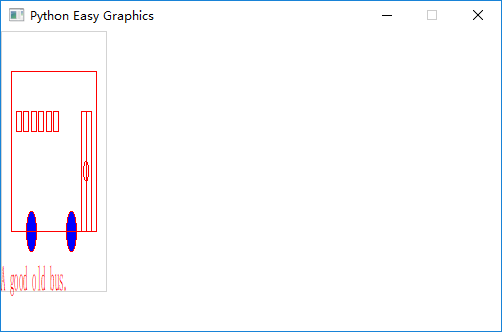## 错切（Shear Mapping）¶

### 沿X轴方向错切¶

```from easygraphics import *
import draw_bus

def main():
init_graph(500, 300)

shear(0.5, 0)
draw_bus.draw_bus()

pause()
close_graph()

easy_run(main)
```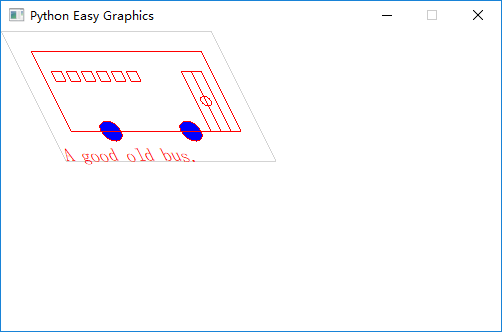### 沿Y轴方向错切¶

```from easygraphics import *
import draw_bus

def main():
init_graph(500, 300)

shear(0, 0.5)
draw_bus.draw_bus()

pause()
close_graph()

easy_run(main)
```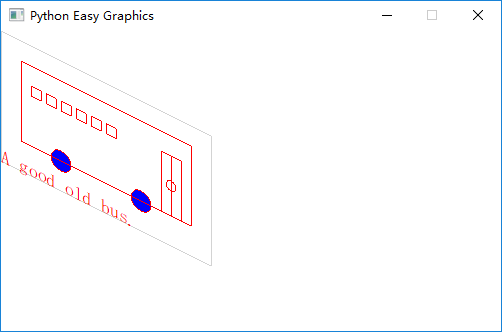### 同时沿两轴错切¶

```from easygraphics import *
import draw_bus

def main():
init_graph(500, 300)

shear(0.5, 0.5)
draw_bus.draw_bus()

pause()
close_graph()

easy_run(main)
```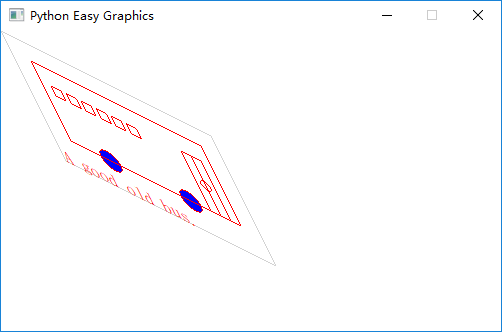## 反射（Reflection）¶

### 以垂直线为轴反射¶

```from easygraphics import *
import draw_bus

def main():
init_graph(500, 300)
reflect(105, 0, 105, 1)
draw_bus.draw_bus()
pause()
close_graph()

easy_run(main)
```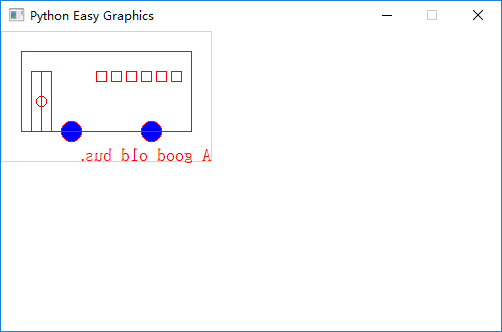### 以水平线为轴反射¶

```from easygraphics import *
import draw_bus

def main():
init_graph(500, 300)

reflect(0, 65, 1, 65)

draw_bus.draw_bus()
pause()
close_graph()

easy_run(main)
```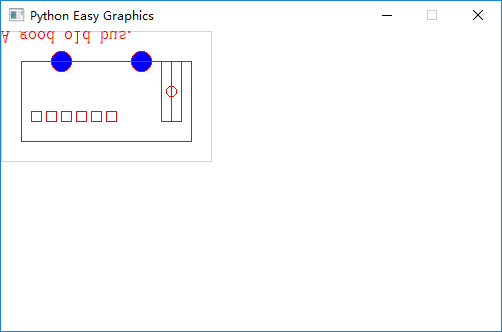### 以任意直线为轴反射¶

```from easygraphics import *
import draw_bus

def main():
init_graph(500, 300)

draw_bus.draw_bus()

set_color("gray")
set_line_style(LineStyle.DASH_LINE)
line(0, 300, 500, 0)
set_line_style(LineStyle.SOLID_LINE)

reflect(0, 300, 500, 0)
draw_bus.draw_bus()
pause()
close_graph()

easy_run(main)
```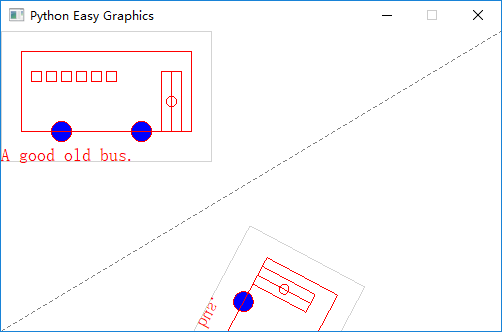## 复合变换¶

```from easygraphics import *
import draw_bus

def main():
init_graph(500, 300)

# move the origin to the center of the image
translate(250, 150)

# rotate around the bus center
translate(105, 65)
rotate(180)
translate(-105, -65)

# shear arount the bus center
translate(105, 65)
shear(0.5, 0.5)
translate(-105, -65)

# scale
scale(1.2, 1.2)
draw_bus.draw_bus()
pause()
close_graph()

easy_run(main)
```## 在Y轴向上的坐标系中绘图¶

Easygraphics专门提供了set_flip_y()函数，来处理这种情况（只翻转图片，不翻转文字)。

```from easygraphics import *
import draw_bus

def main():
init_graph(500, 300)

translate(250, 150)
translate(105, 65)
rotate(-45)
translate(-105, -65)

set_flip_y(True)
# reflect(1,0)

translate(105, -65)
shear(0.2, 0.2)
translate(-105, 65)

draw_bus.draw_bus()
set_color("blue")
draw_rect_text(0, 0, 210, 130, "This is a very good day!")
pause()
close_graph()

easy_run(main)
```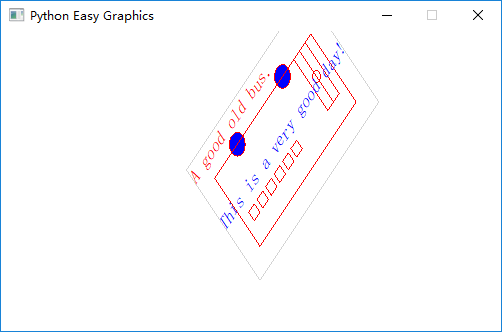```from easygraphics import *
import draw_bus

def main():
init_graph(500, 300)

translate(250, 150)
translate(105,65)
rotate(-45)
translate(-105,-65)

reflect(1,0)

translate(105, -65)
shear(0.2,0.2)
translate(-105, 65)

draw_bus.draw_bus()
set_color("blue")
draw_rect_text(0,0,210,130,"This is a very good day!")
pause()
close_graph()

easy_run(main)
```